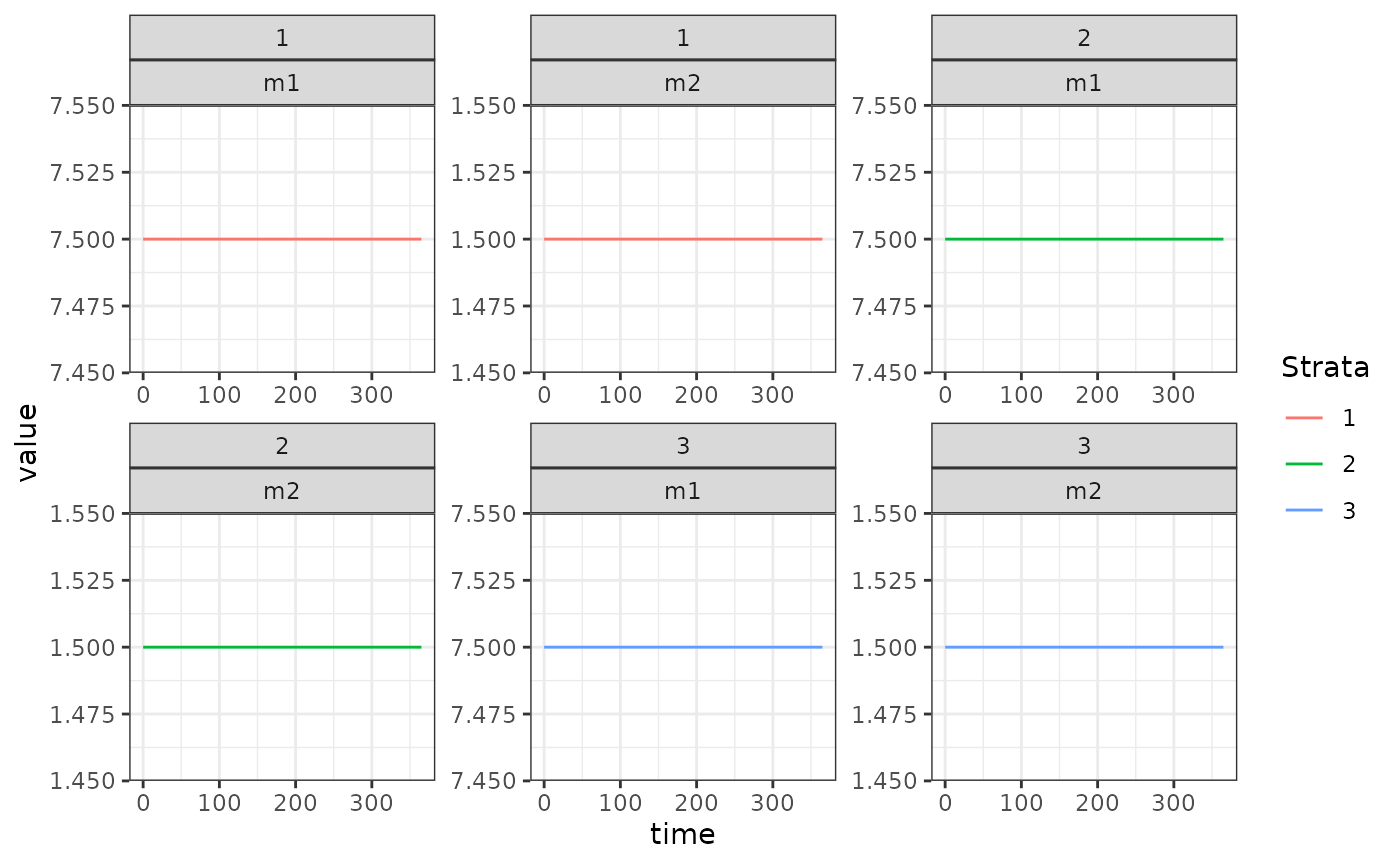This is a hybrid model which tracks the mean multiplicity of infection (superinfection) in two compartments. The first, $$m1$$ is all infections, and the second $$m2$$ are apparent (patent) infections. Therefore $$m2$$ is “nested” within $$m1$$. It is a “hybrid” model in the sense of Nåsell (1985).

## Differential Equations

The equations are as follows:

$\dot{m_{1}} = h - r_{1}m_{1}$ $\dot{m_{2}} = h - r_{2}m_{2}$ Where $$h = b EIR$$, is the force of infection. Prevalence can be calculated from these MoI values by:

$x_{1} = 1-e^{-m_{1}}$ $x_{2} = 1-e^{-m_{2}}$ The net infectious probability to mosquitoes is therefore given by:

$x = c_{2}x_{2} + c_{1}(x_{1}-x_{2})$

Where $$c_{1}$$ is the infectiousness of inapparent infections, and $$c_{2}$$ is the infectiousness of patent infections.

## Equilibrium solutions

One way to proceed is assume that $$m_{2}$$ is known, as it models the MoI of patent (observable) infections. Then we have:

$h = r_{2}/m_{2}$ $m_{1} = h/r_{1}$ We can use this to calculate the net infectious probability, and then $$\kappa = \beta^{\top} \cdot x$$, allowing the equilibrium solutions of this model to feed into the other components.

## Example

library(exDE)
library(deSolve)
library(data.table)
library(ggplot2)

Here we run a simple example with 3 population strata at equilibrium. We use exDE::make_parameters_X_hMoI to set up parameters. Please note that this only runs the human population component and that most users should read our fully worked example to run a full simulation.

We use the null (constant) model of human demography ($$H$$ constant for all time). While $$H$$ does not appear in the equations above, it must be specified as the model of bloodfeeding ($$\beta$$) relies on $$H$$ to compute consistent values.

## The Long Way

Here, we build a model step-by-step.

nStrata <- 3
H <- c(100, 500, 250)
residence = rep(1,3)
searchWtsH = rep(1,3)
b <- 0.55
c1 <- 0.05
c2 <- 0.25
r1 <- 1/250
r2 <- 1/50
TaR <- matrix(data = 1, nrow = 1, ncol = nStrata)

m20 <- 1.5
h <- r2*m20
m10 <- h/r1

EIR <- h/b
params <- make_parameters_xde()
params$nStrata = nStrata params$nPatches = 1

params$eir = rep(EIR, 3) F_eir = function(t, pars){ pars$eir
}

params = make_parameters_demography_null(pars = params, H=H, residence=residence, searchWts=searchWtsH, TaR=TaR)

params = make_parameters_X_hMoI(pars = params, b = b, c1 = c1, c2 = c2, r1 = r1, r2 = r2)
params = make_inits_X_hMoI(pars = params, rep(m10, nStrata), rep(m20, nStrata))
params = make_indices(params)
y0 <- get_inits(params)

out <- deSolve::ode(y = y0, times = c(0, 365), func = xDE_diffeqn_cohort,
parms = params, method = 'lsoda', F_eir = F_eir)
out1 <- out

colnames(out)[params$Xpar$m1_ix+1] <- paste0('m1_', 1:params$nStrata) colnames(out)[params$Xpar$m2_ix+1] <- paste0('m2_', 1:params$nStrata)

out <- as.data.table(out)
out <- melt(out, id.vars = 'time')
out[, c("Component", "Strata") := tstrsplit(variable, '_', fixed = TRUE)]
out[, variable := NULL]

ggplot(data = out, mapping = aes(x = time, y = value, color = Strata)) +
geom_line() +
facet_wrap(Strata ~ Component, scales = 'free') +
theme_bw()## Using Setup

Xo = list(b=0.55, c1=0.05, c2=0.25, r1=1/250, r2=1/50, m20=1.5)
h = with(Xo, r2*m20)
Xo$m10 = with(Xo, h/r1) Hpop = c(100, 500, 250) h/b #>  0.05454545 fF_eir1 = function(EIR){return(function(t, pars){EIR})} F_eir1 = fF_eir1(EIR) xde_setup_cohort("test_hMoI", F_eir1, "hMoI", HPop=Hpop, Xopts = Xo) ->test_hMoI xde_solve(test_hMoI, 365, 365)$outputs\$deout -> out2
approx_equal(out2, out1)
#> logical(0)